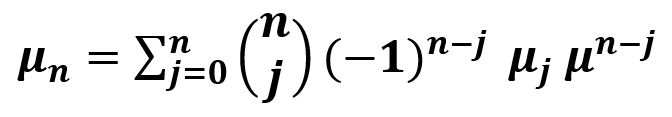Related Articles

# scipy stats.moment() function | Python

• Last Updated : 11 Feb, 2019

`scipy.stats.moment(array, axis=0)` function calculates the nth moment about the mean for a sample i.e. array elements along the specified axis of the array (list in python).

Its formula –Parameters :
array : Input array or object having the elements to calculate the moment.
axis : Axis along which the moment is to be computed. By default axis = 0.
moment : Order of central moment that is returned.

Returns : n-th central moment of the array elements based on the set parameters.

Code #1:

 `# Moment``from` `scipy ``import` `stats``import` `numpy as np `` ` `arr1 ``=` `np.array([[``1``, ``31``, ``27``, ``13``, ``21``, ``9``],``                ``[``8``, ``12``, ``8``, ``4``, ``7``, ``10``]]) ``  ` ` ` `print``(``"Oth moment : \n"``, stats.moment(arr1, moment ``=` `0``)) `

Output :

```Oth moment :
[1. 1. 1. 1. 1. 1.]```

Code #2: With multi-dimensional data

 `# Moment ``from` `scipy ``import` `stats``import` `numpy as np `` ` `arr1 ``=` `[[``1``, ``3``, ``27``], ``        ``[``3``, ``4``, ``6``], ``        ``[``7``, ``6``, ``3``], ``        ``[``3``, ``6``, ``8``]]  `` ` `print``(``"Oth moment : \n"``, stats.moment(arr1, moment ``=` `0``))`` ` `print``(``"\n6th moment : \n"``, stats.moment(arr1, moment ``=` `6``))`` ` `print``(``"\n9th moment : \n"``, stats.moment(arr1, moment ``=` `9``, axis ``=` `None``))`` ` `print``(``"\n12th moment : \n"``, stats.moment(arr1, moment ``=` `12``, axis ``=` `1``))`` ` `print``(``"\n10th moment : \n"``, stats.moment(arr1, moment ``=` `10``, axis ``=` `1``))`

Output :

```Oth moment :
[1. 1. 1.]

6th moment :
[5.20609375e+02 9.13256836e+00 4.26392850e+06]

9th moment :
55265909588.26437

12th moment :
[1.53284936e+14 1.63654317e+02 8.83474172e+03 5.17842143e+04]

10th moment :
[5.53094361e+11 6.10464868e+01 1.64971407e+03 7.65588508e+03]```

Attention geek! Strengthen your foundations with the Python Programming Foundation Course and learn the basics.

To begin with, your interview preparations Enhance your Data Structures concepts with the Python DS Course. And to begin with your Machine Learning Journey, join the Machine Learning – Basic Level Course

My Personal Notes arrow_drop_up